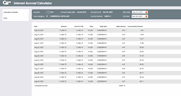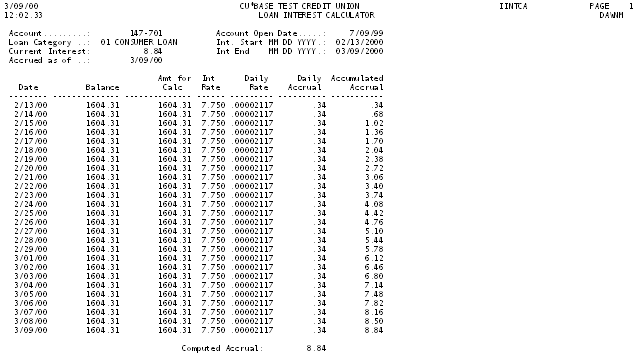# Loan Interest Accrual Calculator

 Screen ID:  Screen Title:  Panel Number: Report Name:IINTCA-01 Interest Accrual Calculator 5097 IINTCAClick here to magnify

This screen appears when Interest Calc (F11) is used on the Member Account Inquiry screen for a loan account.

The Loan Interest Accrual Calculator displays a “what if” calculation showing how each day's interest accrual is calculated using the current configuration parameters. It is NOT a historical representation of what actually happened with daily interest accruals for any given period, but rather serves as a “check and balance” for keeping an eye on how daily interest due amounts are calculated.

·     Remember that only loans configured with interest calculation type “0” (365-day) or “4” (360/365 daily) can be displayed here; an error message will appear when viewing loans with other calculation types.

The top portion of the screen shows current information about the account. The bottom portion of the screen is the calculated sample. By default, the calculation starts as of the day after the last payment was made, and continues through the current date (most recent accrual). A cumulative total is shown for the entire period since the last payment. Use the fields at the top of the screen to change the date range shown.

Assuming you are checking calculations for the period of time since the last payment was made, the Current Interest and Computed Accrual amounts should match. If they do not, further investigation may be needed.

You may enter any period for which transaction history exists for this loan. Keep in mind, however, that the calculator will use only the current rate from the loan account record (MEMBER5 or MEMBER6) when calculating accruals. If this is a variable rate loan product, and the rate was changed at some point during the period being viewed, this calculator will not take that rate change into account.

## Special Note Regarding Variable Rate and Tiered Rate Loans

The Loan Interest Accrual Calculator uses only the current rate when calculating the accrual amounts. This rate is taken directly from the individual loan account record (MEMBER5 or MEMBER6) at the time the calculator is used.

For loans that have Variable Rates, the Calculator will still use the current rate from the loan record, and will not reflect changes made to the variable rate during the life of the loan.

For line-of-credit loans that use the Tiered Rates feature for calculating payments, the process that calculates the payment amount on the loan will also update the loan account record with the appropriate rate according to the loan balance. Therefore, since the rate used by the Calculator will always be taken from the loan account itself, this rate will match the rate that was assigned to the loan the last time a payment was recalculated using Tiered Rates.

## Field Descriptions

 Field Name Description Account Open Date The date this member account was opened. The Balance column will show 0.00 for each date prior to this open date. Current Interest This shows the actual amount of interest currently accrued on this member account. Accrued as of This shows the last date on which accruals were calculated. By default, the Calculator will show sample accrual amounts starting the day after the last payment, up to and including this date. Start End Use these field to enter the period you wish to verify. The Start date defaults to the day after the most recent payment; the End date defaults to the last day on which accruals were calculated (the current date). Both can be changed to view any period since the loan was opened. Calculations can only be performed if transaction history exists, so your credit union's transaction history retention will determine how far back calculations can be displayed. Date This column shows each individual date for which accruals are being calculated. Balance This column shows the actual member balance for each day in the period, determined using transaction history for this member account. Amt for Calc This column shows the amount that was used in the accrual calculation. This will be the same as the Balance column. Rate This column shows the rate that was used to calculate each sample daily accrual. Daily Rate This column shows the daily rate used in the sample accrual calculation. This is determined by dividing the Rate by the number of days in the year (365 or 366 for calc. type 0, 360 for calc. type 4). Daily Interest This column shows the calculated daily interest accrual amount for each day in the selected period.  Remember that this is a calculated rate, not actual history from this member's account. Accumulated Interest This column shows a cumulative accrual amount for each day in the selected period, calculated by adding the Daily Interest amount, one day at a time. Computed Accrual This grand total adds all of the amounts shown in the Daily Interest column for the selected period.

## Buttons

 Buttons Description This key should be used to review the parameters used by CU*BASE when calculating accruals for this loan type. Print (F7) This key can be used to print a report showing the accrual calculations for the selected period. The multiple-page report will be sent directly to your spool file.  To access the report and print it do the following: Use the Escape key to access the Time out window. Click the Work with Spool Files button to access your spool file.  In the Option column on the line of the report enter a 2.  (2 means print).  Use Enter. Enter your printer name in the Printer field and use Enter.  The report will print to the printer you selected. Up arrow (F7) Use this key to exit the calculator and return to the Account Inquiry screen.

## Report Sample

This report will be automatically sent to your default printer when F6-Print is used (the report will begin printing after the Calculator screen has been exited).